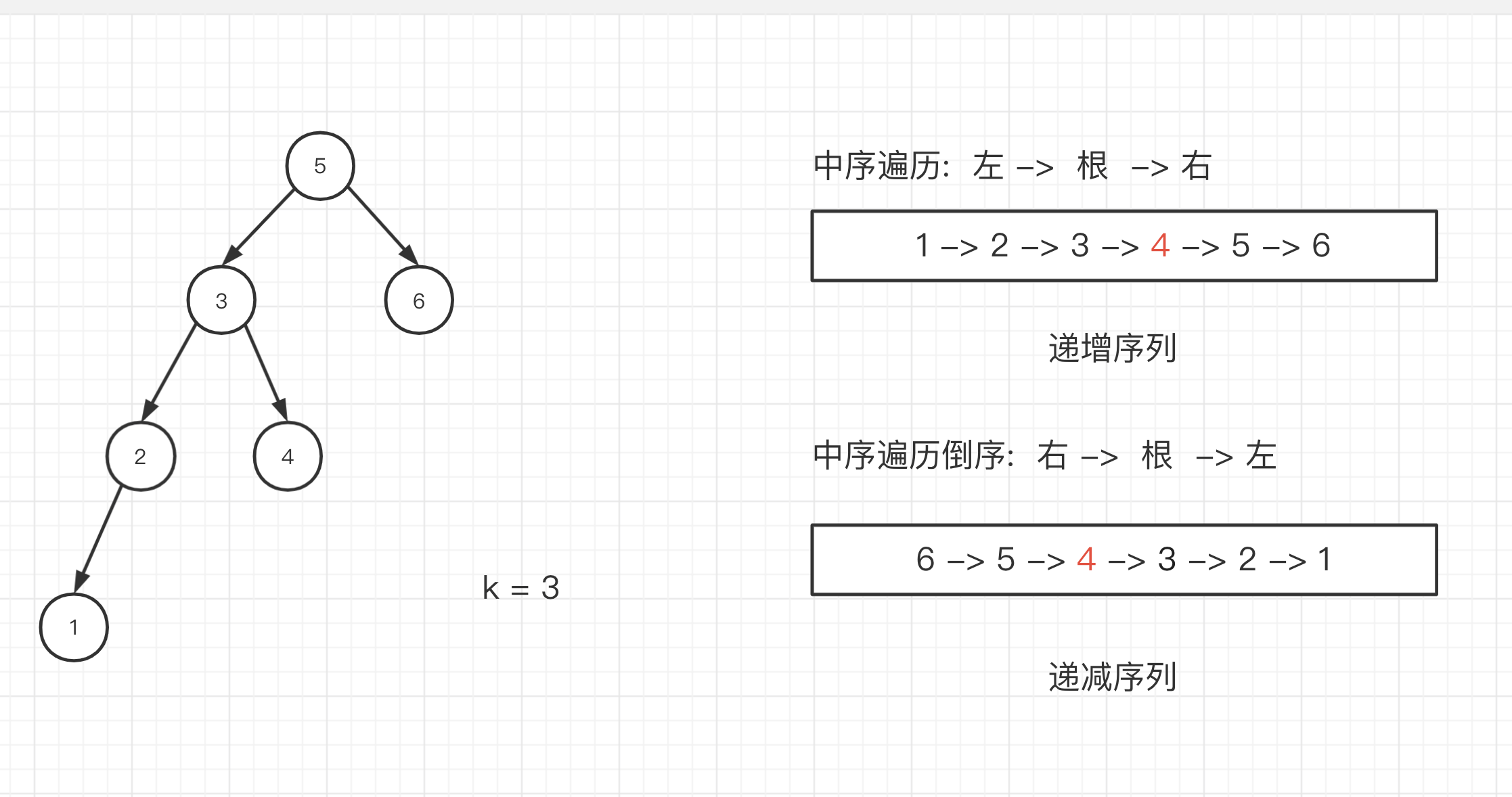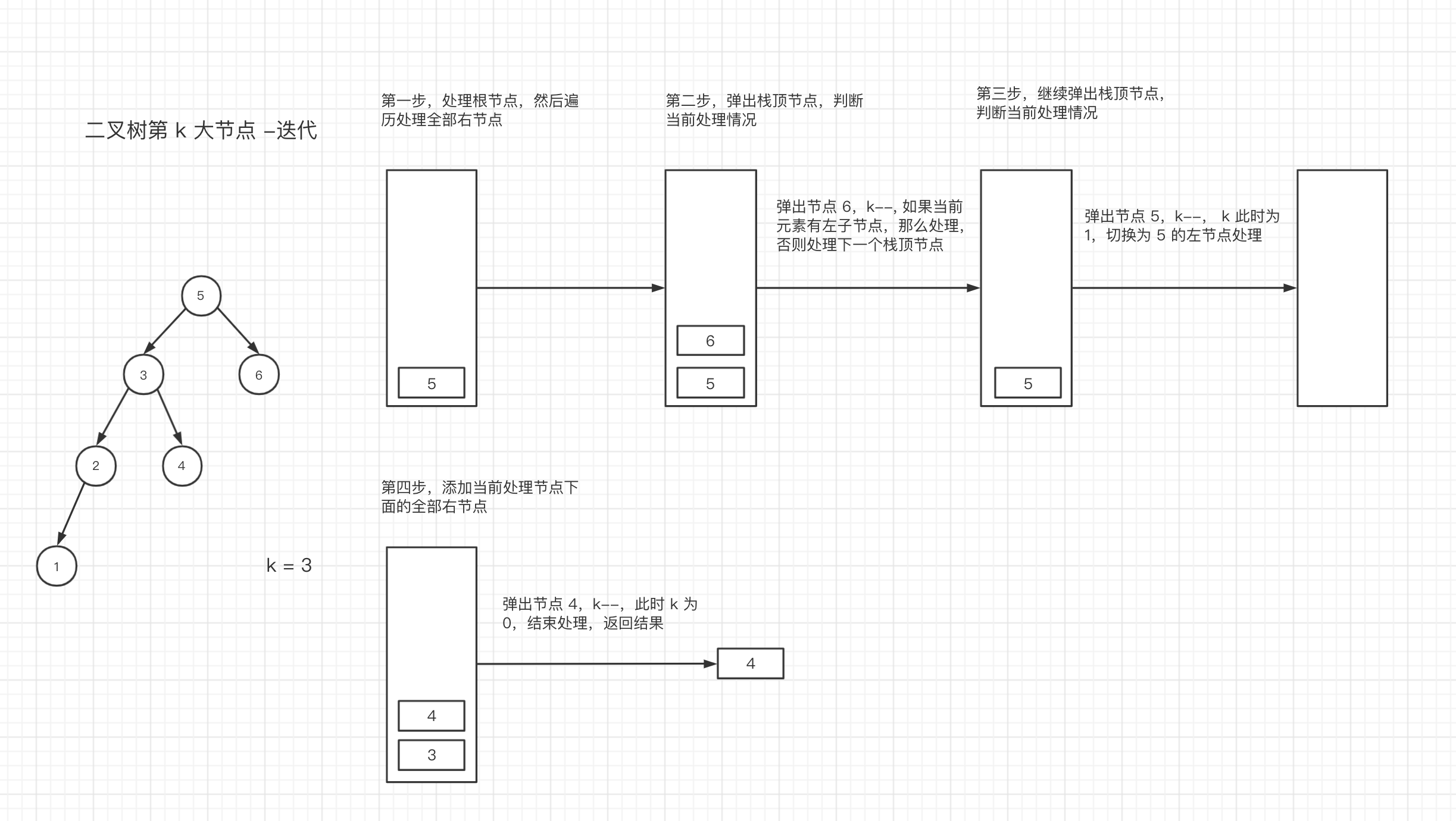# 二叉搜索树的第 k 大的节点

## 题目描述​

``输入: root = [3,1,4,null,2], k = 1   3  / \ 1   4  \   2输出: 4``

``输入: root = [5,3,6,2,4,null,null,1], k = 3       5      / \     3   6    / \   2   4  / 1输出: 4``

## 解法一： 递归​``/** * Definition for a binary tree node. * function TreeNode(val) { *     this.val = val; *     this.left = this.right = null; * } *//** * @param {TreeNode} root * @param {number} k * @return {number} */const kthLargest = (root, k) => {  let res = null; // 初始化返回值  // 因为需要倒序第 k 个，所以处理是右节点，根节点，然后左节点  const dfs = (root) => {    if (!root) return; // 如果当前节点为 null，本轮处理结束    dfs(root.right); // 开始处理右节点    if (k === 0) return; // k 值 为 0，代表已经处理的节点超过目标节点，本轮处理结束    if (--k === 0) {      // 当 k 值 减 1 为 0，表示已经到了我们想要的 k 大 节点，保存当前值      res = root.val;    }    dfs(root.left); // 处理左节点  };  dfs(root); // 从初始化节点开始处理  return res;};``

### 复杂度分析：​

• 时间复杂度 O(N)：无论 k 的值大小，递归深度都为 N，占用 O(N) 时间。
• 空间复杂度 O(N)：无论 k 的值大小，递归深度都为 N，占用 O(N) 空间。

## 解法二： 迭代​``/** * Definition for a binary tree node. * function TreeNode(val) { *     this.val = val; *     this.left = this.right = null; * } *//** * @param {TreeNode} root * @param {number} k * @return {number} */var kthLargest = function (root, k) {  if (!root) return 0;  // 声明储存栈  const stack = [];  // 判断当前栈否有节点和当前遍历节点位置  while (stack.length || root) {    while (root) {      // 往栈里添加当前节点，同时切换为右节点处理      stack.push(root);      root = root.right;    }    // 取出当前栈顶元素，根据添加的顺序，当前元素是栈内最大的    const cur = stack.pop();    k--;    if (k === 0) return cur.val;    // 切换为左节点处理    root = cur.left;  }  return 0;};``
• 时间复杂度 O(N)：需要遍历整棵树一次，复杂度为 O(N)
• 空间复杂度 O(N)：需要额外空间栈进行储存树，复杂度为 O(N)

1. 剑指 offer